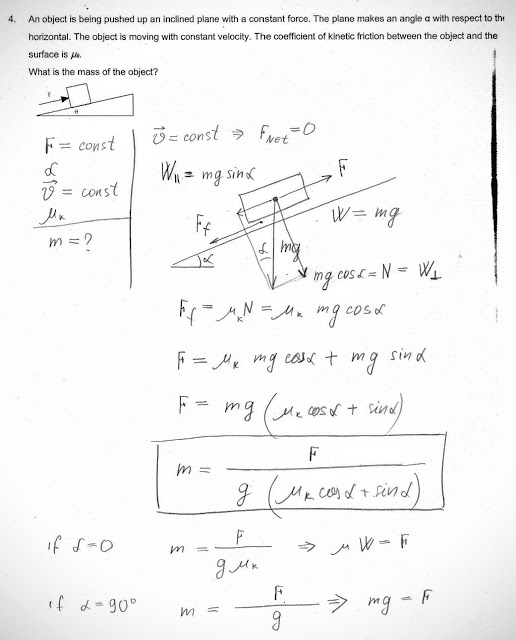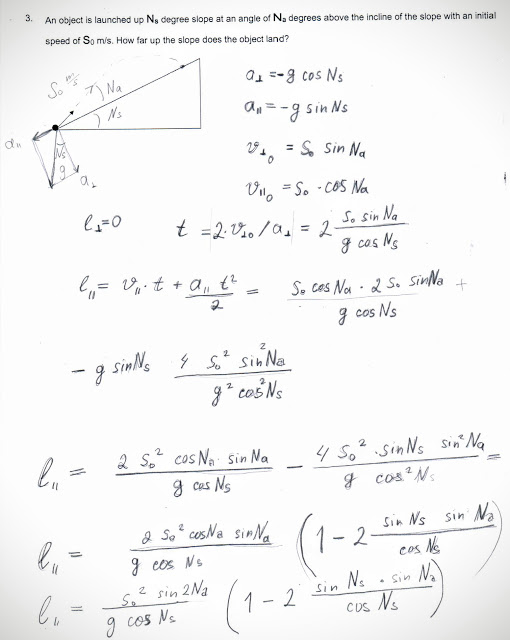## Saturday, April 25, 2015

### An object is being pushed up an inclined plane with a constant force F

 An object is being pushed up an inclined plane with a constant force F. The plane makes an angle θ with respect to the horizontal. The object is moving with constant velocity. The coefficient of kinetic friction between the object and the surface is µk. What is the mass of the object?From Calculus-Based Physics I: Problems with Solutions

### An object is launched up Ns degree slope at an angle of Na degrees above the incline of the slope

 An object is launched up Ns degree slope at an angle of Na degrees above the incline of the slope with an initial speed of S0 m/s. How far up the slope does the object land?From Calculus-Based Physics I: Problems with Solutions

### A ball of mass M is thrown from the ground at an angle of α from the vertical with a speed S

A ball of mass M is thrown from the ground at an angle of α from the vertical with a speed S. The ball lands on top of a building that height is h. What is the speed of the ball just before it lands on the roof of the building?From Calculus-Based Physics I: Problems with Solutions

### A car moving along the x direction has acceleration, ax that varies with time

A car moving along the x direction has acceleration, ax that varies with time as shown in the figure. At the moment, t = 0 s, the car is located at x0 = 1.0 m and has a velocity v0 of 2.0 m/s in the positive x direction. What is the position of the car at t = 3.0 s?

## Thursday, April 16, 2015

### What is Magnitude of Angle between Vectors?

The vector A = (Ax , Ay) = ( +2.0 m, +2.0 m) and the vector B = (Bx , By) = ( +1.0 m, -1.0 m).
What is the magnitude of the angle between the vectors A and B?